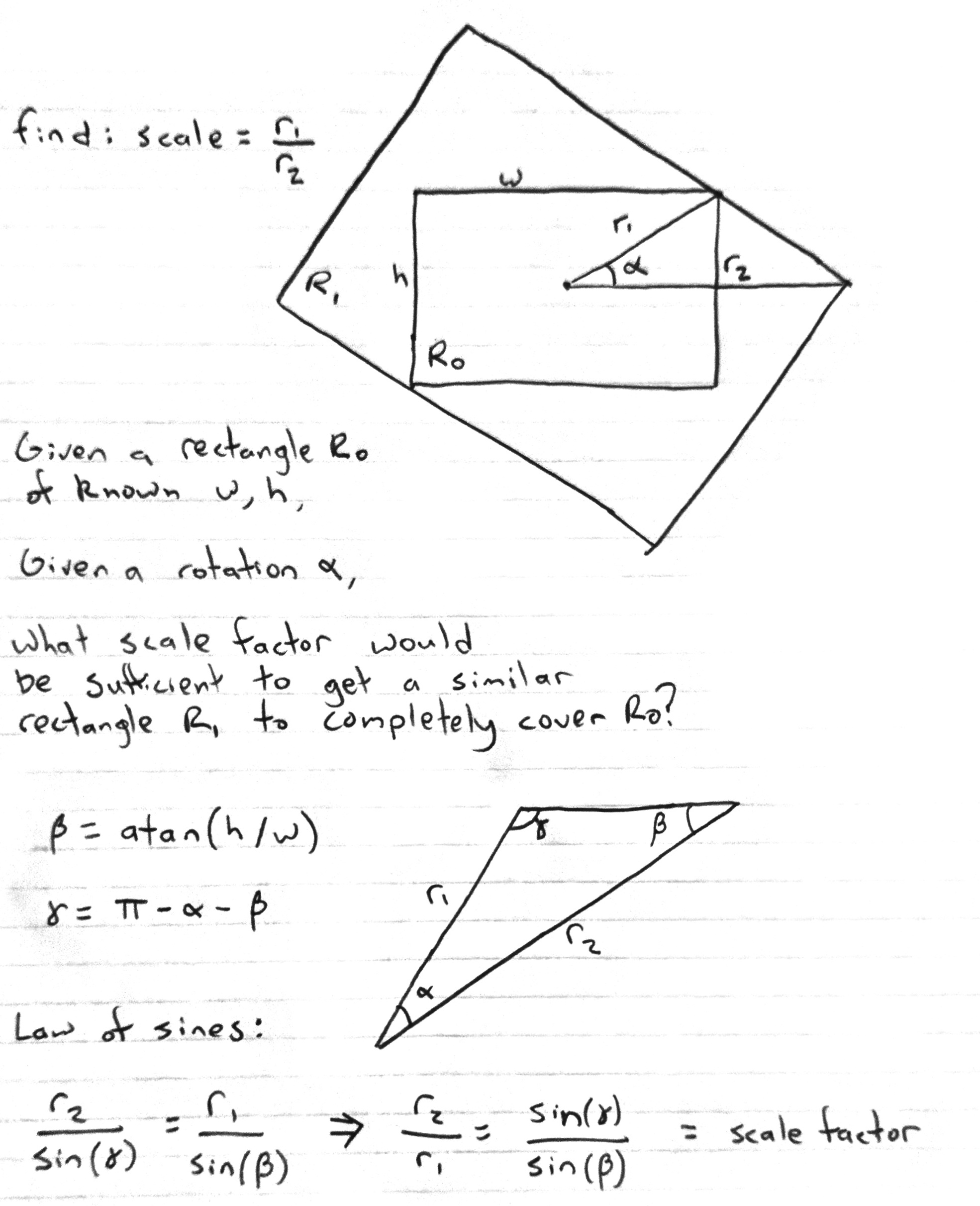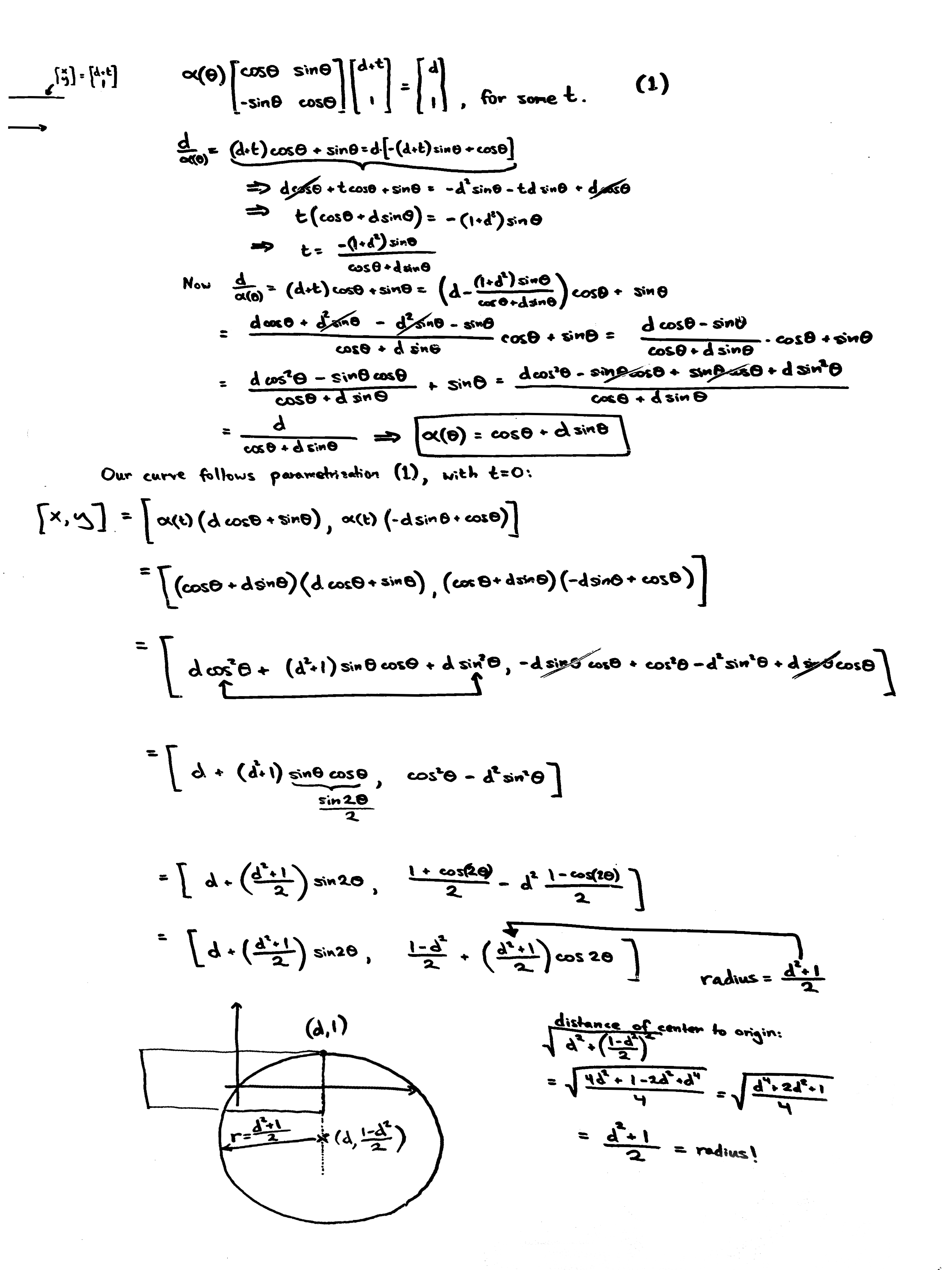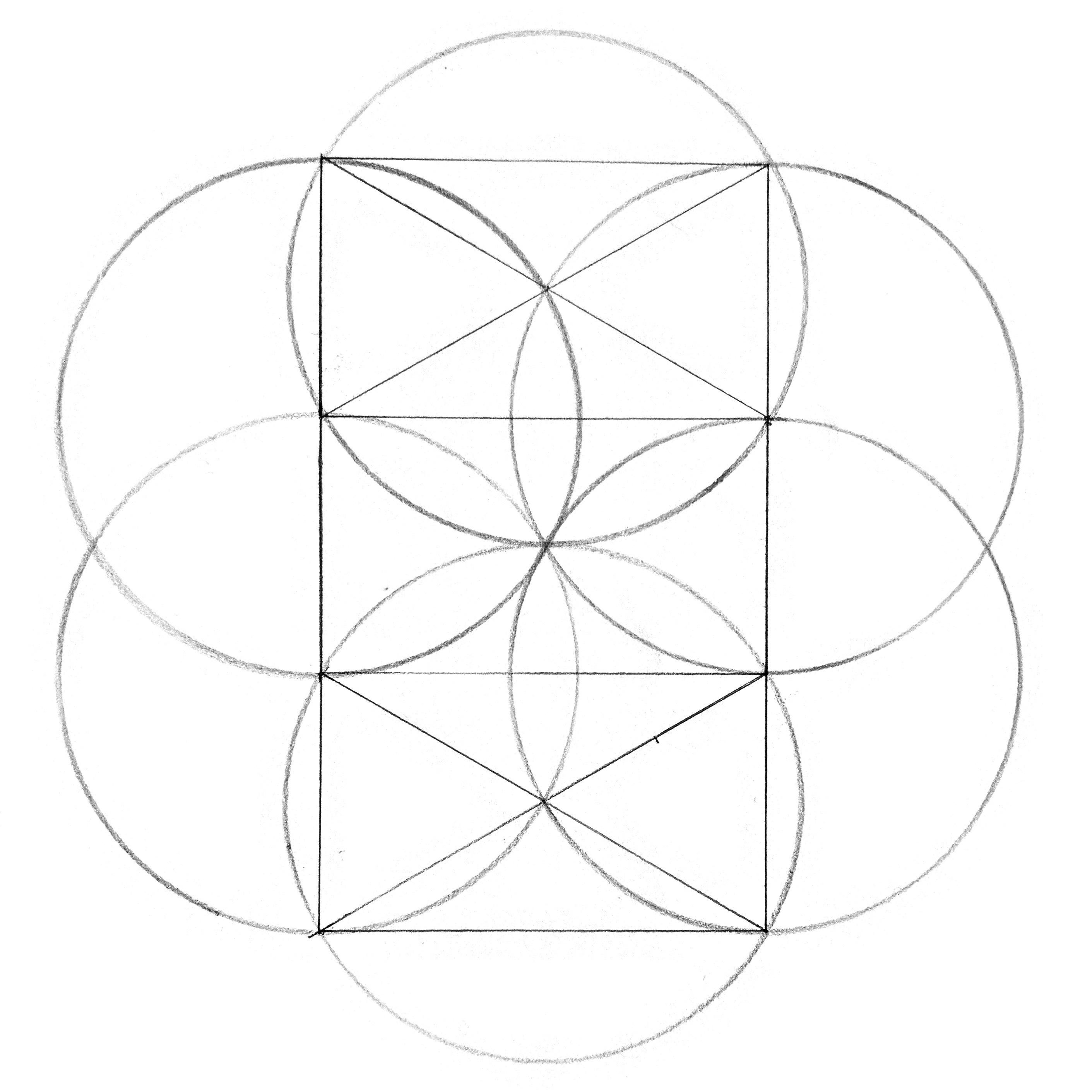## Rotate and Circumscribe

Take a fixed rectangle, and a similar, concentric rectangle which circumscribes the original rectangle as it rotates about the common center. How does the circumscribing rectangle scale as a function of angle?

I came upon this fun little geometry problem recently, and something caught me by surprise. It's the question of just what is the shape of the path that the corners of the scaling, rotating rectangle trace out.

### Context

I needed to correct for some videos (image sequences) I'd shot where the camera isn't quite level. They're 1920x1080 and I want to rotate the image by a few degrees, but I don't want any black/empty spots in any corners leftover. So given a certain rotation, I then want to scale up the content by the minimum amount necessary such that the imagery fills the original 1920x1080 area. Unfortunately, this doesn't seem to be a built-in option in either FFMPEG or Pillow which I'm using for my Deanimation work.

This drawing shows how I worked out the scale factor, with fairly straightforward trigonometry:### The surprise

After working out the formula for the scale as a function of angle, I then went to animate it using Processing. For fun, I tried rendering the above animation without clearing the background every frame (so that the rectangles would stick around and accumulate, as if leaving tracks behind) and I was struck by what I saw.

Perhaps you'd like to take a moment before proceeding and see if you can envision what happens? Can you imagine shape that the outer, circumscribing rectangle traces out? Do you expect to see something like well defined lobes? If so, what kind of curvature might the lobes have? When you're ready, play the following video and see what happens.

Upon watching this animate for the first time, what really surprised me was seeing that the path is comprised of circular arcs. Circular arcs! Who would've guessed?

### Derivation of the trajectories

I shared my findings with my friend Dan Walsh. I presented the problem and told him I was pretty convinced the arcs are circular, just from seeing the trace of the animation. In my mind, we tend to have good eyes for when something is circular.

Dan did one better. He worked out a parametric expression for the trace of a corner of the circumscribing rectangle and showed that it is indeed a circular arc. Fantastic.Dan also created the following beautiful interactive plot with Desmos allowing you to vary the proportions of the fixed rectangle and see the resulting envelope.

### Where have I seen this before?

Contemplating this, Dan and I both had a strong feeling of Déjà vu. I had seen something similar a long time ago, where something like a rectangle moves and slides and magically traces out a circle. After sleeping on it, it came to me the next morning. It was the wonderful "draw a circle with a framing square and two nails" trick from carpentry.

Watching this demonstration again after many years, I find it absolutely beautiful. I can recognize it as a manifestation of the inscribed angle theorem I learned in high school. Yet somehow learning it as a theorem in school is one thing – it is quite impressive that an angle, inscribed in a semicircle across a diameter always comes out to 90-degrees. But this, seeing a circle generated from a sliding 90-degree angle feels more magical, even though it's kind of the straightforward inverse.

It's fun to imagine which knowledge came first in ancient history, and whether one might have influenced the other:

1. The geometer/mathematician "inscribed angles in a semicircle equal 90-degrees"
2. The carpenter/builder "a square swept across two nails traces out a semicircle"

### Check out the code for yourself

Here's a sketch done in processing. (I used the desktop Processing app to locally produce the animations in this article with the same code and minimal tweaks.)

### Further investigation:

#### Q: What is the point at which the envelope has six symmetric lobes?

My original sketch used a 16:9 rectangle, thanks to starting from the common video format. And the resulting envelope wass pretty close to a hexagonally symmetric arrangement of six lobes. But what would be the exact proportion (if any) that would produce six equal lobes in a regular hexagon arrangement?

I had a hunch it would be the golden ratio (because my 16:9 ratio from my original images, which is close to the golden ratio made something so close to six equal lobes). And when you set the proportions to 1:1.618 it looks pretty convincing, but if you measure the resulting figure it's clearly off. It turns out there is such a proportion, and it is not the golden ratio, it is the square root of 3.

### A: Square root of 3

I have Dan to thank for determining the critical proportion is not the golden ratio, but rather the square root of 3. That is the proportion seen in the first animations in this article.

It is fairly simple to see for yourself. Take a fixed rectangle of proportions 1:√3 and then draw the circumscribing rectangle at 90-degrees. The resulting arrangement is equilavent to having three root-3 rectangles stacked on top of one another. In general, any rectangle of proportions 1:sqrt(n) is comprised of n similar rectangles by dividing the original rectangle along the long edge.The circular arcs have radius equal to the unit edge of the rectangle. Four of the arcs are centered at the four corners of the fixed rectangle. The other two arcs are centered at the midpoints of the upper and lower rectangles.

A root-3 rectangle's proportions match naturally with a regular hexagon. The short side correspondes with one side of the hexagon, and the long side with the minor diameter of the hexagon. The well-known 30-60-90 triangle (of side lengths 1:2:√3 comprises half of a root-3 rectangle.

You can see that when you draw the diagonals of a root-3 rectangle, you end up with a pair of equalateral triangles point to point. The root-3 rectangle is my new favorite rectangle. I'm glad it's not the golden rectangle yet again.

### More fun

And finally, here's more animations where a whole bunch of rectangles are nested iteratively, each one circumscribing the next and scaling appropriately as the angle varies.

In this one, the inner rectangle rotates at a constant speed and each successive rectangle is offset from the previous by an equal amount:

In this one, the inner rectangle rotates randomly using Perlin noise, and each successive rectangle performs the same rotation but delayed a bit, which produces a lovely propagation behavior.